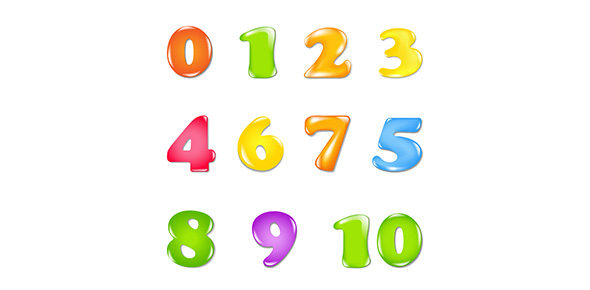# Extending The Number Line

Approved & Edited by ProProfs Editorial Team
The editorial team at ProProfs Quizzes consists of a select group of subject experts, trivia writers, and quiz masters who have authored over 10,000 quizzes taken by more than 100 million users. This team includes our in-house seasoned quiz moderators and subject matter experts. Our editorial experts, spread across the world, are rigorously trained using our comprehensive guidelines to ensure that you receive the highest quality quizzes.
| By NChavez2016
N
NChavez2016
Community Contributor
Quizzes Created: 1 | Total Attempts: 425
Questions: 6 | Attempts: 442SettingsIn this quiz the knowledge represented will include: negative numbers, positive numbers, and number line ends and placing. This quiz will help people to identify positive and negative numbers and where they need to be placed on a number line?

• 1.

### The number line has 2 positive ends?

• A.

True

• B.

False

B. False
Explanation
The number line does not have positive ends. It extends infinitely in both the positive and negative directions. Therefore, it does not make sense to say that it has "positive ends".

Rate this question:

• 2.

### Where would -4 go on a number line?

• A.

Right of 0

• B.

Left of 0

• C.

On the middle

B. Left of 0
Explanation
The number -4 would go to the left of 0 on a number line because it is a negative number. In a number line, positive numbers are placed to the right of 0 and negative numbers are placed to the left of 0. Since -4 is a negative number, it would be located to the left of 0.

Rate this question:

• 3.

### Is zero a negative or a positive number?

• A.

Negative

• B.

Positive

• C.

None

C. None
Explanation
Hint: It goes in the middle of the number line!

Rate this question:

• 4.

### Which of these numbers are opposite?

• A.

3,3

• B.

-3,3

• C.

0,3

B. -3,3
Explanation
The numbers -3 and 3 are opposite because they have the same magnitude (absolute value) but different signs. In other words, one is positive and the other is negative. This is a common way to define opposite numbers in mathematics.

Rate this question:

• 5.

### Numbers greater than 0 are positive?

• A.

True

• B.

False

A. True
Explanation
Numbers greater than 0 are considered positive because positive numbers are any numbers greater than 0. In mathematics, positive numbers are values that are greater than zero and can be represented on the positive side of the number line. Therefore, any number greater than 0 is considered positive.

Rate this question:

• 6.

### Which number has a lower value?

• A.

-300

• B.

-500

• C.

Both have the same value

B. -500
Explanation
Hint: Which is further away from 0?

Rate this question:

Related TopicsBack to top